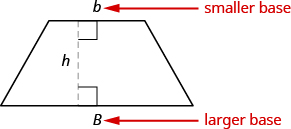## Skills Review for Numerical Integration

### Learning Outcomes

• Complete a table values with solutions to an equation
• Use sigma (summation) notation to calculate sums and powers of integers
• Find the area of a trapezoid

In the Numerical Integration section, we will estimate the value of definite integrals using a variety of estimation rules. Here we will review some essential topics that will help us to better understand the various estimation rules.

## Complete a Table of Function Values

A table of values can be used to organize the y-values or function values that result from plugging specific x-values into a function’s equation.

Suppose we want to determine the various values of the equation $f(x)=2x - 1$ at certain values of x. We can begin by substituting a value for x into the equation and determining the resulting value of the function. The table below lists some values of x from –3 to 3 and the resulting function values.

 $x$ $f(x)=2x - 1$ $-3$ $f(-3)=2\left(-3\right)-1=-7$ $-2$ $f(-2)=2\left(-2\right)-1=-5$ $-1$ $f(-1)=2\left(-1\right)-1=-3$ $0$ $f(0)=2\left(0\right)-1=-1$ $2$ $f(2)=2\left(2\right)-1=3$ $3$ $f(3)=2\left(3\right)-1=5$

We can look for trends among our function values by looking at the table. For example, in this case, as x-values increase, so do the y-values. Also, it seems reasonable to assume, based on the table, an x-value of 1 would result in a function value of 1. You can verify this for yourself by plugging 1 into $f(x)=2x - 1$.

### Example: Completing a table of function values

Create a table of function values for $f(x)=-x+2$. Use various integers from -5 to 5 as the x-values you plug into the function.

## Use Sigma (Summation) Notation

Summation notation (also known as summation notation) is used to make it easier to write lengthy sums. The Greek capital letter $\Sigma$, sigma, is used to express long sums of values in a compact form. For example, if we want to add all the integers from 1 to 20 without sigma notation, we have to write

$1+2+3+4+5+6+7+8+9+10+11+12+13+14+15+16+17+18+19+20$.

We could probably skip writing a couple of terms and write

$1+2+3+4+\cdots+19+20$,

which is better, but still cumbersome. With sigma notation, we write this sum as

$\displaystyle\sum_{i=1}^{20} i$,

which is much more compact.

Typically, sigma notation is presented in the form

$\displaystyle\sum_{i=1}^{n} a_i$

where $a_i$ describes the terms to be added, and the $i$ is called the index. Each term is evaluated, then we sum all the values, beginning with the value when $i=1$ and ending with the value when $i=n$. For example, an expression like $\displaystyle\sum_{i=2}^{7} s_i$ is interpreted as $s_2+s_3+s_4+s_5+s_6+s_7$. Note that the index is used only to keep track of the terms to be added; it does not factor into the calculation of the sum itself. The index is therefore called a dummy variable. We can use any letter we like for the index. Typically, mathematicians use $i$, $j$, $k$, $m$, and $n$ for indices.

### A General Note: Summation Notation

The sum of the first $n$ terms of a series can be expressed in summation notation as follows:

$\sum _{i=1}^{n}{a}_{i}$

This notation tells us to find the sum of ${a}_{i}$ from $i=1$ to $i=n$.

$k$ is called the index of summation, 1 is the lower limit of summation, and $n$ is the upper limit of summation.

### Example: EXpanding Summation Notation

Evaluate $\sum _{i=3}^{7}{i}^{2}$.

### Try It

Evaluate $\sum _{i=2}^{5}\left(3i - 1\right)$.

## Find the Area of a Trapezoid

A trapezoid is a four-sided figure, a quadrilateral, with two sides that are parallel and two sides that are not. The parallel sides are called the bases. We call the length of the smaller base $b$ and the larger base $B$. The height, $h$, of a trapezoid is the distance between the two bases as shown in the image below.The formula for the area of a trapezoid is:

$Area_{trapezoid}=\dfrac{1}{2}h(b+B)$

### Example: Finding the Area of a Trapezoid

Find the area of a trapezoid whose height is 6 inches and whose bases are 14 and 11 inches.